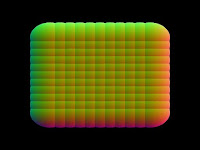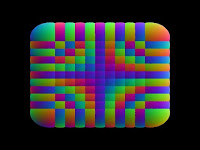## 10/27/07

### Shaders to render normals : NOT!A useful debugging tool is to get a colour representation of the normals in a scene. With a bit of maths, it can also produce some nice, free colouring for an intro. Above is a correct image. It shows a whole bunch of spheres. Red is the x component of the normal, green the y and blue is z. Study it and you'll see its right. Only it took me a few hours to get this right. I made a very simple error, which maybe others do too so I thought I'd document it here.

Hmmm, this is what I actually got:Pretty,yes. Wrong, definitely. Here is the WRONG CODE. This code has a serious bug:
`const GLchar *normalsvsh="\varying vec3 n;\void main(){\n = normalize(gl_Normal*gl_NormalMatrix);\gl_Position=ftransform();\}";const GLchar *normalsfsh="\varying vec3 n;\void main(){\gl_FragColor = vec4((n+1.0)*0.5,1);\}";`
Can you spot the error? Heres a clue, the spheres are rotating about their centres at different speeds.

The solution is...
`n = normalize(gl_NormalMatrix*gl_Normal);\`

#### 2 comments:

1.n = normalize ( gl_Normal * gl_NormalMatrix );

is incorrect code! The correct code is:

n = normalize ( gl_NormalMatrix * gl_Normal );

Matrix maths isn't commutative of course and I forgot about this in my shaders.

2.Anonymous30/10/07

This comment has been removed by a blog administrator.